# Building Mutual Fund Portfolio

In today’s India, investing in traditional fixed income means like Fixed Deposits, National Savings Certificates (NSC), etc. can no longer provide for future requirements of an individual. As in early 2018, rate of interest in Fixed Deposits from Leading Private Sectors Banks is around 6.75% for 1 year investment and about 6% for 10 years investment. Government of India taxes Fixed Deposit income.

Rate of Interest from NSC is 7.8% as on date.

Mutual Funds provide an alternative investment method which has the potential to provide much higher rate of return. However, as the investment includes investing in Stock Market, this investment is subject to higher amount of risk. Experts have observed that in spite of the ups and downs of the Stock Market, if an investor stays invested in Mutual Funds for about 8 years, risk is almost zero.

Having said this, it is important to note that it takes careful planning to build a winning Mutual Fund Portfolio. I provide a method for this planning.

The general rule of investing is that the portfolio should be diversified. Diversification reduces the risk of the portfolio. As experts have said that “Do not put all the Eggs in one Basket”. Similarly, while building the Mutual Fund portfolio, it should be taken care that the portfolio is diversified.

Based on generic information available, Mutual Funds can be chosen from Equity Funds, Debt Funds and Balanced Funds. Equity Funds are Mutual Funds where the majority of the investment is in Equity or the Stock Market. These funds generally carry a higher amount of risk and at the same time are expected to provide higher amount of returns. Debt Funds invest majority of the money in Debt instruments. These funds are much less volatile and carry much lower level of risk while providing for much lower rate of return. Balance Funds have almost equal investments in Equities and Debt Instruments.

Equity Funds can be further classified by investment style i.e. whether the funds is invested in Large Caps or Medium Caps or Small Caps. Large Caps generally carry lower level of risks, Medium Caps generally carry higher level of risk than Large Caps and Small Caps generally carry significantly higher levels of risk.

We start the discussion with the assumption that based on the above considerations and other considerations, I have decided to invest Rs. 5,00,000 in 5 Mutual Funds as shown in the below table.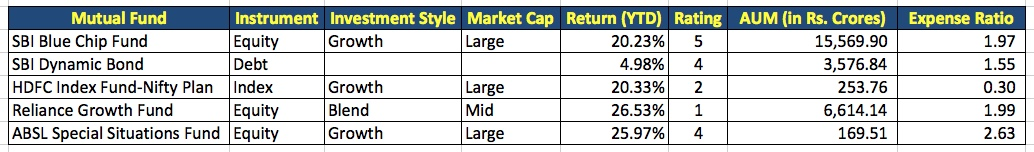I have chosen funds from 4 different Fund Houses. Also, I have chosen funds invested in Equity, Debt and also one Index Fund. The Equity Funds are invested in Large Caps and Mid Caps. The AUM shows the current Fund Size. Expense Ratio is generally lower for Index Funds as these Funds follow the Index.

We can mathematically find out if our portfolio is diversified or not. To do this, we need to find out the returns from each of the Funds over the last 7 to 10 years. I collected this information from Economic Times website. These figures may vary at the time when you read this article.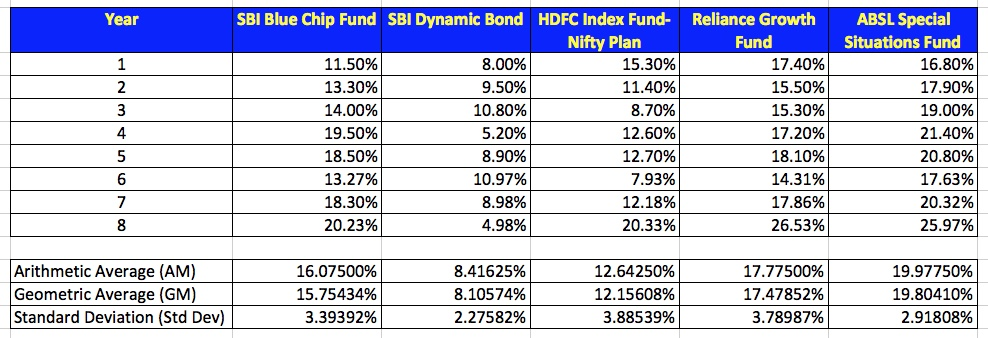I have used Excel Functions to calculate the Arithmetic Average, Geometric Average and Standard Deviation.

The Standard Deviation indicates the level of risk in the Fund. The Averages can be considered as a decent expected return in the future; though this is not guaranteed.

Next, we need to find the Correlations between the Funds. Before we can find the Correlations, we need to determine the Covariances. Covariance can be found using the Excel Function COVAR. The result is as shown below.Having calculated the Covariances, we can find the Correlation using the formula below.

`Correlationxy = Covariancexy / (Standard Deviationx * Standard Deviationy)`

The calculated Correlations are shown below.We find that none of the Funds are very highly correlated with each other. While this is not the ideal portfolio, however, it is a decently diversified portfolio. This is just an example for illustration and NOT any form of advice for investment.

Consider that we have decided to invest Rs. 5,00,000 in these 5 funds. Next we need to decide how much funds to invest in each fund.

We will study 3 different approaches to determine this.

1. First we will determine the fund allocation in each Mutual Fund as per our Risk Appetite. Risk Appetite can be determined by calculating the Beta for each Fund and then the Beta for the Portfolio.
2. Next, we will determine the fund allocation in each Mutual Fund as per the Sharpe Ratio of the Portfolio. Sharpe Ratio provides a measure of Risk-to-Variability in the investment.

## How to decide Fund Allocation based on the Beta for the Portfolio?

Beta is a measure of the level of risk in the Portfolio. We can adjust the Beta for our Portfolio based on our Risk Profile. Different levels of Beta will provide for different allocation to the individual Mutual Funds in the Portfolio.

To determine the Beta for the Portfolio, we first need to find the Beta for each Mutual Fund. Using formula from Capital Asset Pricing Model (CAPM), we can determine Beta for each Mutual Fund using the formula below.

`Return on Asset (Ra) = Risk Free Rate (Rf) + [Rate of Return from Market (Rm) - Risk Free Rate (Rf)] * Beta`

We can rearrange the above formula to solve for Beta as follows.

`Beta = (Ra - Rf) / (Rm - Rf)`

Risk Free Rate is the rate we would expect to get from investments which are nearly risk free. We could consider Fixed Deposits in Banks to be such investments. So, in our calculations, we will consider Risk Free Rate (Rf) = 6%.

Rate of Return from Market would vary from Investor to Investor. For Investor who have a lot of investment in Stock Market, the Rate of Return from Market would be Rate at which the Market has been giving returns i.e. around 15% at the time of writing. For Investors, who majorly invest in Fixed Deposits, NSCs, Kisan Vikas Patras (KVP), Public Provident Fund, etc. , we could consider Rate of Return from Market as about 8%. For this illustration, we will consider Rate of Return from Market (Rm) = 15%.

We will consider the Arithmetic Average of Returns for each Mutual Fund as the Return on Asset (Ra).

So, we get the following values for the Beta for each Mutual Fund.Having determined the Beta for the individual Mutual Funds, we can determine the Beta for the Portfolio by multiplying the individual Betas with the proportions of the Funds Allocated to each Mutual Fund. Suppose we initially decide that we will allocate equal proportions in each Mutual Fund i.e. Rs. 1,00,000 in each Mutual Fund or 20% in each Mutual Fund. Then, in Excel, we can use the function SUMPRODUCT to determine the Portfolio Beta.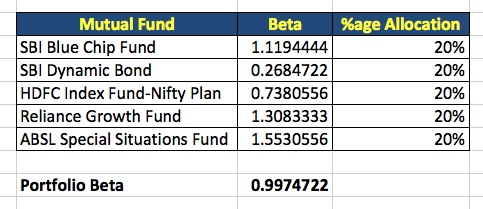If the above Fund allocation be our decision, then based on the Arithmetic Average Rate of Return for each Mutual Fund, the rate of return from the Portfolio over a 10 year period would be 15.59% with Standard Deviation of the Portfolio being 2.086%. However, this may not be the best allocation of Funds in each Mutual Fund.

The Rate of Return for the determined as follows:

1. Calculate the returns from each Mutual Fund as per the Arithmetic Average Rate of Return for the respective Mutual Fund at the end of 10 years.
2. Add all the 5 values obtained in step 1.
3. Use the RATE function in Excel to determine the Rate of Return for the Portfolio.

It takes a lot more effort to determine the Standard Deviation for the Portfolio. Suppose the individual Standard Deviations of each Mutual Fund are S1, S2, S3, S4 and S5. Suppose the proportion of funds allocated to each Mutual Fund is p1, p2, p3, p4 and p5. Then the formula for calculating the Standard Deviation of the Portfolio is as follows:

`Standard Deviation of Portfolio = SQRT( S12 * p12 + S22 * p22 + S32 * p32 + S42 * p42 + S52 * p52 + 2 * S1 * S2 * p1 * p2 + 2 * S1 * S3 * p1 * p3 + 2 * S1 * S4 * p1 * p4 + 2 * S1 * S5 * p1 * p5 + 2 * S2 * S3 * p2 * p3 + 2 * S2 * S4 * p2 * p4 + 2 * S2 * S5 * p2 * p5 + 2 * S3 * S4 * p3 * p4 + 2 * S3 * S5 * p3 * p5 + 2 * S4 * S5 * p4 * p5 )`

We will apply Linear Programming to determine the fund allocation. Linear Programming problems can be solved in Excel using the Add-In Solver.

For setting up the Linear Programming Problem, we need the Objective Function, Variables and Constraints.

Our Objective Function in this case is to maximise the Beta.

Our Variables are the proportions of funds to allocate to each Mutual Fund.

The Constraints are as follows:

1. The sum total of all the proportions should be 100%.
2. Further, let us constrain that we should not invest more than 35% in any of the Mutual Fund (This can be altered as per one’s preferences of diversification).
3. Lastly, let us set that the Beta should be less than or equal to 1.6 (This can be set as per one’s risk appetite).

The set up in Solver would look like as shown below.On solving this problem, we get the following allocation of Funds in each Mutual Fund.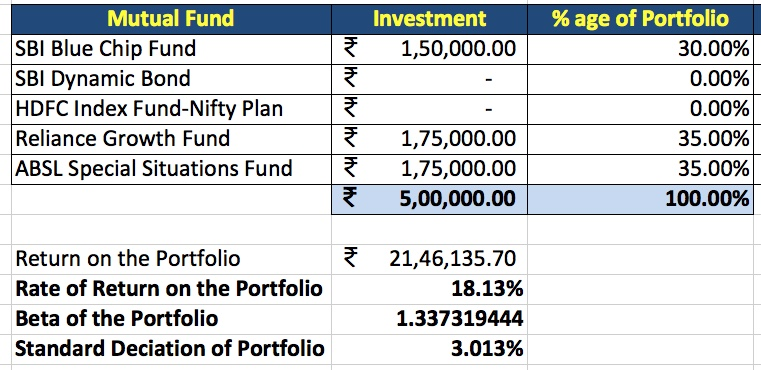Table – A : Fund Allocation to maintain a maximum Beta of 1.6

We notice that the Rate of Return has increase while the Standard Deviation has also increased. So, this portfolio can deliver better returns at a higher level of risk.

Suppose, we conclude that this is too risky for us. We could recalculate by reducing the level of Beta to 1.3. We have to change the Solver configuration as follows.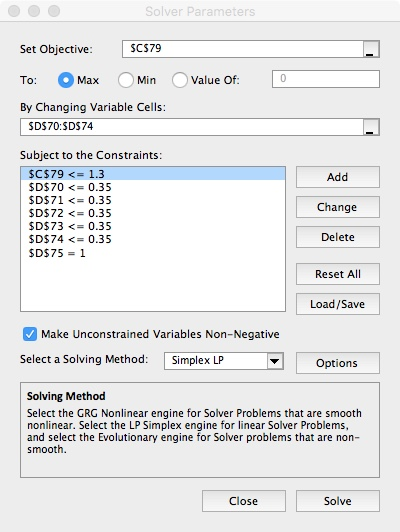On solving, we get the following allocation.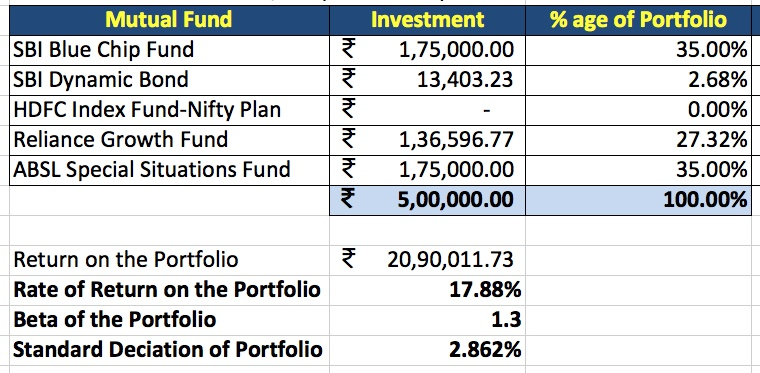Table – B : Fund Allocation to maintain a maximum Beta of 1.3

## How to decide Fund Allocation based on the Sharpe Ratio for the Portfolio?

Sharpe Ratio can be calculated using the formula below.

`Sharpe Ratio = (Return on Asset - Risk Free Rate) / Standard Deviation`

Based on Fund Allocation as per Table B, the Sharpe Ratios are calculated as shown below.Now, higher the Sharpe Ratio, the Portfolio return is higher. So, we see the Fund Allocation such that Sharpe Ratio for the Portfolio is maximised. The Solver needs setting up as shown below.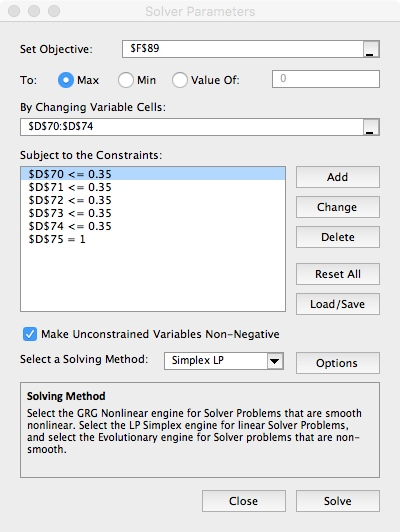The Fund Allocation is as follows.Table – C : Fund Allocation to maintain a maximum Sharpe Ratio

We notice that the Fund Allocation has not changed from Table B. But this may not be the case all the time.

Categories: Others

This site uses Akismet to reduce spam. Learn how your comment data is processed.